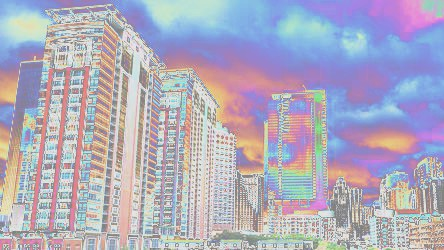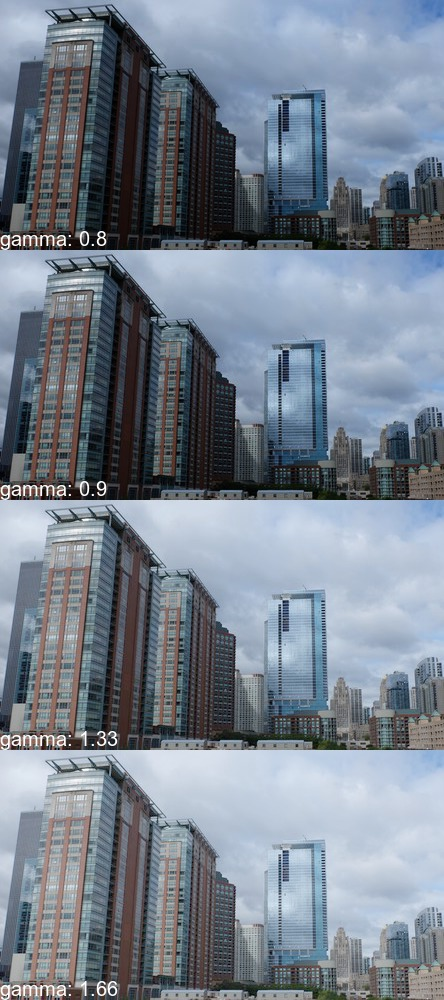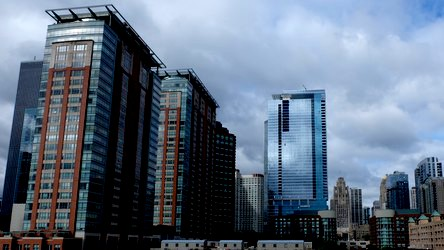Color Enhancement¶

Evaluate Expression¶

New in version 0.4.1.

Pixel channels can be manipulated by applying an arithmetic, relational, or logical expression. See `EVALUATE_OPS` for a list of valid operations.

For example, when given image `enhancement.jpg`:We can reduce the amount of data in the blue channel by applying the right-shift binary operator, and increase data in the right channel with left-shift operator:

```from wand.image import Image

with Image(filename='enhancement.jpg') as img:
# B >> 1
img.evaluate(operator='rightshift', value=1, channel='blue')
# R << 1
img.evaluate(operator='leftshift', value=1, channel='red')
```Function Expression¶

New in version 0.4.1.

Similar to `evaluate()`, `function()` applies a multi-argument function to pixel channels. See `FUNCTION_TYPES` for a list of available function formulas.

For example, when given image `enhancement.jpg`:We can apply a Sinusoid function with the following:

```from wand.image import Image

with Image(filename='enhancement.jpg') as img:
frequency = 3
phase_shift = -90
amplitude = 0.2
bias = 0.7
img.function('sinusoid', [frequency, phase_shift, amplitude, bias])
```FX Expressions¶

New in version 0.4.1.

FX special effects are a powerful “micro” language to work with. Simple functions & operators offer a unique way to access & manipulate image data. The `fx()` method applies a FX expression, and generates a new `Image` instance.

For example, when given image `enhancement.jpg`:We can create a custom DIY filter that will turn the image black & white, except colors with a hue between 195° & 252°:

```from wand.image import Image

fx_filter='(hue > 0.55 && hue < 0.7) ? u : lightness'

with Image(filename='enhancement.jpg') as img:
with img.fx(fx_filter) as filtered_img:
filtered_img.save(filename='enhancement-fx.jpg')
```Gamma¶

New in version 0.4.1.

Gamma correction allows you to adjust the luminance of an image. Resulting pixels are defined as `pixel^(1/gamma)`. The value of `gamma` is typically between 0.8 & 2.3 range, and value of 1.0 will not affect the resulting image.

The `level()` method can also adjust `gamma` value.

For example, when given image `enhancement.jpg`:We can step through 4 pre-configured gamma correction values with the following:

```from wand.image import Image

with Image(filename='enhancement.jpg') as img_src:
for Y in [0.8, 0.9, 1.33, 1.66]:
with Image(img_src) as img_cpy:
img_cpy.gamma(Y)
```Level¶

New in version 0.4.1.

Black & white boundaries of an image can be controlled with `level()` method. Similar to the `gamma()` method, mid-point levels can be adjusted with the `gamma` keyword argument.

The `black` and `white` point arguments are expecting values between 0.0 & 1.0 which represent percentages.

For example, when given image `enhancement.jpg`:We can adjust the level range between 20% & 90% with slight mid-range increase:

```from wand.image import Image

with Image(filename='enhancement.jpg') as img:
img.level(0.2, 0.9, gamma=1.1)
img.save(filename='enhancement-level.jpg')
```